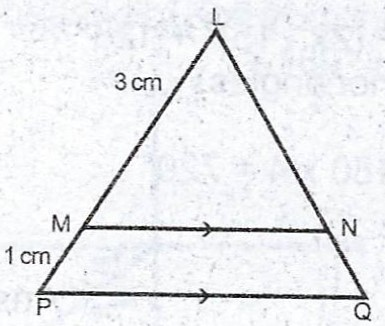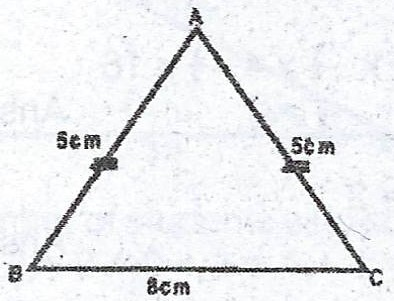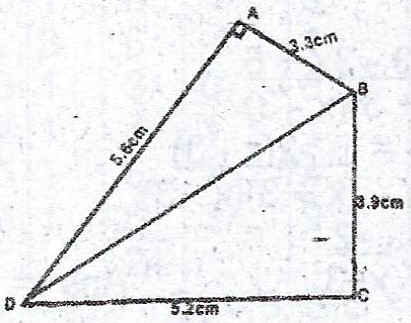### Mathematics Past Questions

4061

(a) Copy and complete the following table of values for the relation $$y = x^{2} - 2x - 5$$

 x -3 -2 -1 0 1 2 3 4 5 y -2 -6 -2 3 10

(b) Draw the graph of the relation $$y = x^{2} - 2x - 5$$; using a scale of 2 cm to 1 unit on the x- axis, and 2 cm to 2 units on the y- axis.

(c) Using the same axes, draw the graph of $$y = 2x + 3$$.

(d) Obtain in the form $$ax^{2} + bx + c = 0$$ where a, b and c are integers, the equation which is satisfied by the x- coordinate of the points of intersection of the two graphs.

(e) From your graphs, determine the roots of the equation obtained in (d) above.

4062

(a) The mean of 1, 2, x, 11, y, 14, arranged in ascending order, is 8 and the median is 9. Find the values of x and y.

(b)In the diagram, MN || PQ, |LM| = 3cm and |LP| = 4cm. If the area of $$\Delta$$ LMN is 18$$cm^{2}$$, find the area of the quadrilateral MPQN.

4063

(a) A surveyor walks 100m up a hill which slopes at an angle of 24° to the horizontal. Calculate, correct to the nearest metre, the height through which he rises.

(b)In the diagram, ABC is an isosceles triangle. |AB| = |AC| = 5 cm, and |BC| = 8 cm. Calculate, correct to the nearest degree, < BAC.

(c) Two boats, 70 metres apart and on opposite sides of a light-house, are in a straight line with the light-house. The angles of elevation of the top of the light-house from the two boats are 71.6° and 45°. Find the height of the light-house. [Take $$\tan 71.6° = 3$$].

4064

(a) A cylindrical well of radius 1 metre is dug out to a depth of 8 metres. (i) calculate, in m$$^{3}$$, the volume of soil dug out ; (ii) if the soil is used to raise the level of rectangular floor of a room 4m by 12m, calculate, correct to the nearest cm, the thickness of the new layer of soil. [Take $$\pi = \frac{22}{7}$$].

(b)The diagram shows a quadrilateral ABCD in which < DAB is a right- angle. |AB| = 3.3 cm, |BC| = 3.9 cm, |CD| = 5.6 cm. (i) find the length of BD. (ii) show that < BCD = 90°.

4065

(a) The first term of an Arithmetic Progression (A.P) is 8. The ratio of the 7th term to the 9th term is 5 : 8. Calculate the common difference of the progression.

(b) A sphere of radius 2 cm is of mass 11.2g. Find (i) the volume of the sphere ; (ii) the density of the sphere ; (iii) the mass of a sphere of the same material but with radius 3cm. [Take $$\pi = \frac{22}{7}$$].